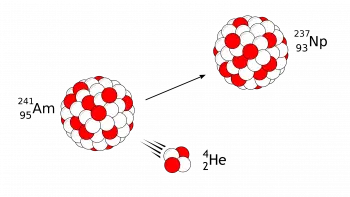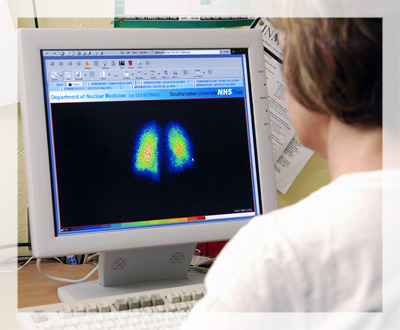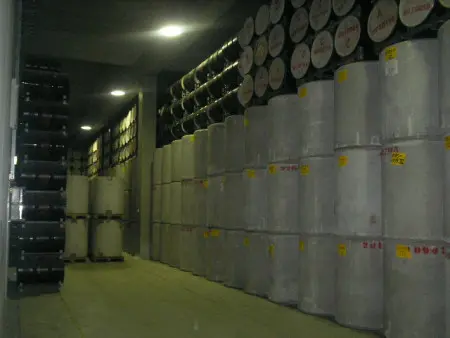# Radioactive Decay: What It Is, Types, Formula and ExamplesRadioactive decay is a physical phenomenon that involves the spontaneous transformation of unstable atomic nuclei into more stable ones.

This nuclear process plays a crucial role in nuclear physics and has significant implications in fields such as medicine and geological dating.

Radioactive decay is a physical process that occurs in atomic nuclei, the central part of an atom that contains protons and neutrons.

The stability of a nucleus depends on the relationship between the nuclear force that holds it together and the electromagnetic force that tends to repel positively charged protons. When this relationship becomes unbalanced and the core becomes unstable, the radioactive decay process comes into play.

Radioactive decay can take several forms, but the three main types are alpha (α), beta (β), and gamma (γ). Each of them involves specific changes in the structure of the nucleus and the release of particles or energy.

### Alpha (α) DecayIn alpha decay, an unstable nucleus emits an alpha particle, which consists of two protons and two neutrons. This particle has a high energy and positive electrical charge, which makes it easily detectable.

A common example of alpha decay is the decay of uranium-238 into thorium-234, releasing an alpha particle in the process.

### Beta (β) Decay

Beta decay involves the transformation of a neutron into a proton or vice versa within the nucleus. When a neutron turns into a proton, it emits a negative beta particle (an electron) and an antineutrino. On the other hand, when a proton turns into a neutron, a positive beta particle (a positron) and a neutrino are emitted.

This process can lead to the conversion of one element to another in the periodic table.

An example of beta decay is the conversion of carbon-14 to nitrogen-14.

### Gamma Decay (γ)

Gamma decay involves the release of electromagnetic radiation in the form of gamma rays. This radiation is highly energetic and can easily penetrate matter, making it a dangerous form of ionizing radiation.

Gamma decay often accompanies other types of decay, such as alpha and beta, to release excess energy from the nucleus.

## Formula and Calculation of Radioactive Decay

The calculation of radioactive decay is based on a mathematical equation that describes how the activity (or amount of radioactive material) of a sample changes over time. This equation is known as the Law of Radioactive Decay. The general form of the radioactive decay equation is as follows:

N(t)=N 0 ·e −λt

Where:

• N(t) is the amount of radioactive material remaining at time t.

• N 0 is the initial amount of radioactive material at time t=0.

• λ is the radioactive decay constant, which is related to the half-life (t ½ ) of the radioactive material by the formula λ=ln⁡(2) / t ½

• t is the elapsed time.

Below I present a table that shows the radioactive decay constant (λ) and the half-life (t ½ ) of some of the main radioactive materials:

 Radioactive material Decay constant (λ) [1/s] Half-life (t ½ ) [years] Uranium-238 (U-238) 1.54×10 −10 4,468×10 9 Potassium-40 (K-40) 5.81×10 −10 1.277×10 9 Carbon-14 (C-14) 1.21×10 −4 5,730 Radium-226 (Ra-226) 2.19×10 −6 1,599 Polonium-210 (Po-210) 4.98×10 −3 138.4 Cesium-137 (Cs-137) 9.23×10 −4 30.2 Strontium-90 (Sr-90) 7.57×10 −4 28.8 Tritium (H-3) 4.92×10 −3 12.3

## Examples and ApplicationsRadioactive decay has a number of important implications in science and technology.

Some of the areas in which knowledge about radioactive decay is or has been used include:

• Nuclear medicine : In nuclear medicine, radioactive isotopes are used for diagnosis and treatment. For example, iodine-131 is used to treat hyperthyroidism, while technetium-99m is used in medical imaging studies such as bone scans.
• Geological dating : Radiocarbon dating, based on the beta decay of carbon-14, allows scientists to determine the age of fossils and archaeological objects.
• Power Generation : Within nuclear power plants, nuclear fission reactions that are triggered within a nuclear reactor to generate electricity involve controlled radioactive decay.
• Radiation Detectors – These devices are based on this physical process and are essential for nuclear safety and radiation protection.

## Drawbacks and ConcernsDespite its many beneficial applications, radioactive decay also presents challenges and concerns.

One of the main problems is the safe management of radioactive waste generated by the nuclear industry. This waste must be stored safely for thousands of years due to its long-term danger.

Additionally, exposure to ionizing radiation can be harmful to human health. Radiation can damage DNA and increase the risk of cancer and other diseases. Therefore, it is crucial to maintain strict control and regulation of radioactive sources and ensure the safety of workers and the general public.

Author:

Published: September 25, 2023
Last review: September 25, 2023